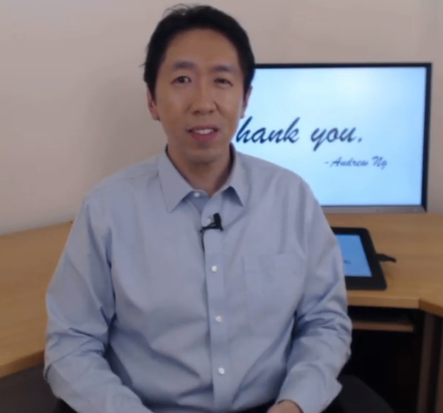# [Sequential Models] week3. Sequence models & Attention mechanism

This week: seq2seq.

## I-Various sequence to sequence architectures

### Basic Models

e.g. Machine translation
encoder network: many-to-one RNN
decoder network: one-to-many RNN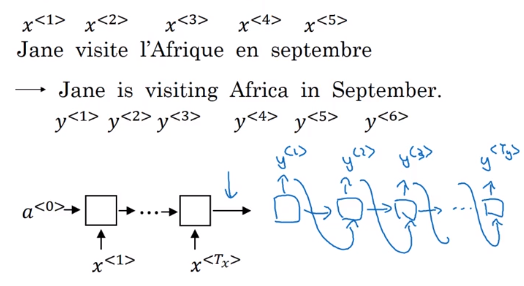This architecture also works for image captioning: use ConvNet as encoder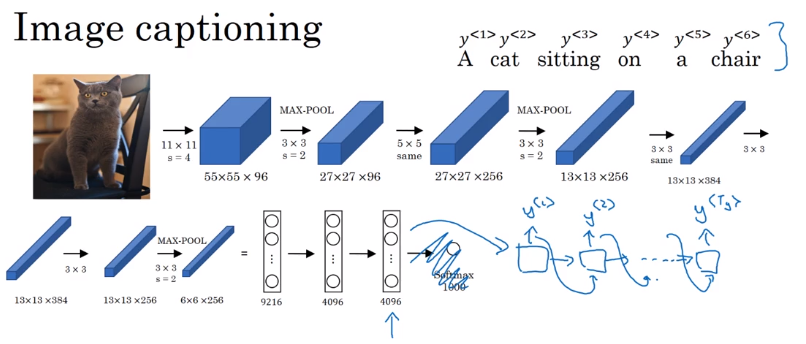Difference between seq2seq and generating new text with language model: seq2seq don't randomly choose a translation, but choose most likely output sequence.

### Picking the most likely sentence

Machine translation (or seq2seq in general): a conditional language model.

• language model: `P(y<1>,...,y<T>)`, `x<i> = y<i-1>`, initial activation = `a<0>`
• seq2seq: feed encoder output as initial activation → `P(y<1>...y<T>|x=input seq)`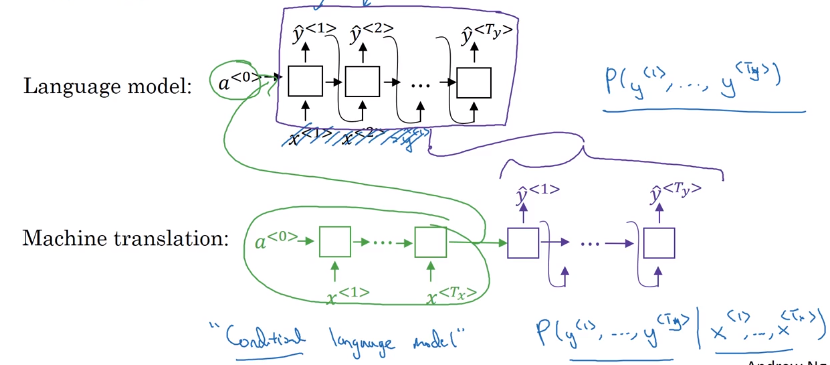Want to sample most likely output sequence (instead of random sampling)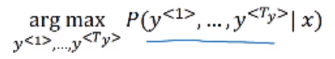• For output sequence of length L, there are |V|^L possiblilities.
• greedy search: pick most likely word at each step → doesn't work well
• approximate(not guaranteed) search algo: beam search (next section).

Approximately find most likely output sequence.
algo
parameter: beam width `B` = 3 (beam serach = greedy for B=1)

• step 1: find `B` most likely choices for first word argmax `P(y<1>|x)`
• step 2: for each of `B` previous choices → compute second word probabilities

→ compuate `P(y<1>, y<2>|x)` by Bayes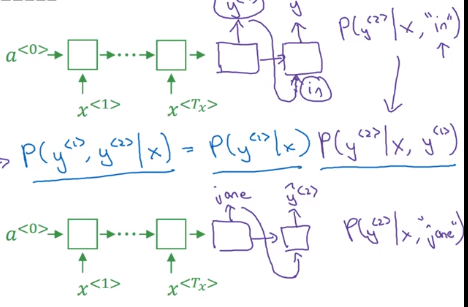initialize B copies of the network, hardwiring each of the B choices of first word from last step
⇒ keep top `B` most likely first 2 words {y<1>,y<2>}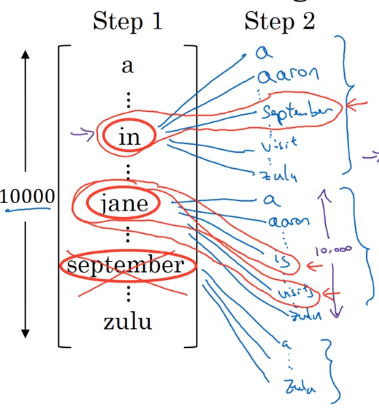• step 3: similar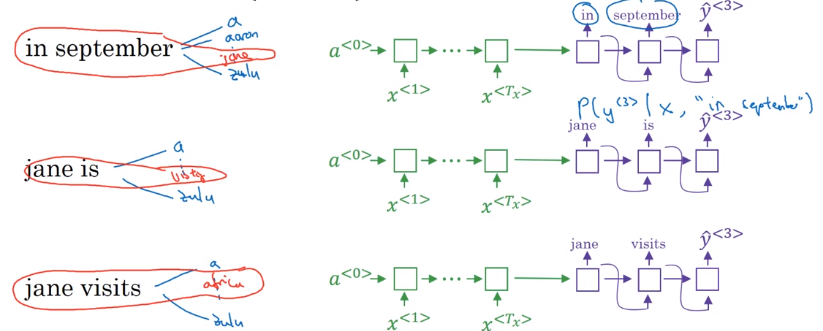Length normalization
original object to optimize:
P(y<1>...y) = product of conditional proba: P(y<1>|x)P(y<2>|y<1>,x)...
(in practice: taking log → sum of log-probas, more numerically stable)
with original object function, tends to prefer shorter output sequences
⇒ normalize the probability by output length, i.e. average proba of each word
"normlized log-likelihood"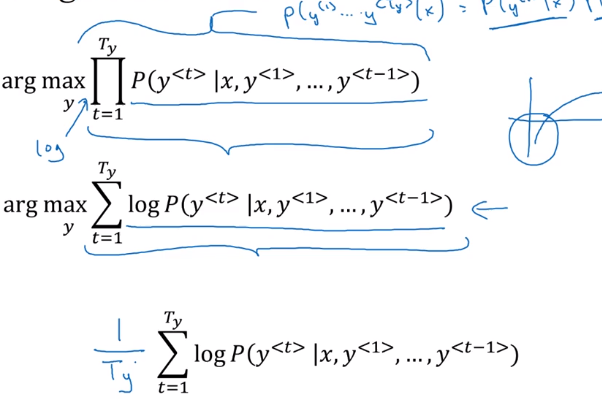In practice: use a softer normalization: normalize by `T^alpha` (typical value: alpha=0.7)

• alpha=1: fully normalizing by length
• alpha=0: no normalization

Beam width choice

• large B: better approximation, better result, slower
• small B: worse result, but faster

In production: B=10
In research: B=~1000

When error occurs: figure out whether it's due to beam search or RNN model.
Given `yhat` and `y*`(human result):
→ feed `yhat` and `y*` to RNN language model, compute the probability of each sequence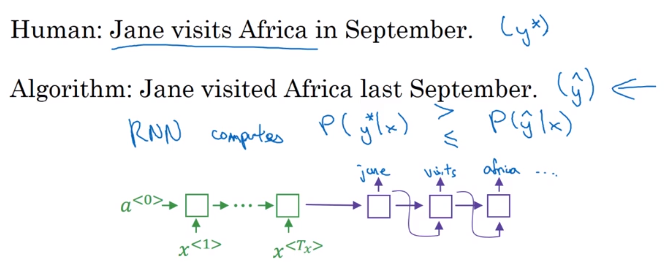• If `P(y*)>P(yhat)`⇒ beam seach needs improvement
• If `P(y*)<P(yhat)` ⇒ RNN needs improvement

### Bleu Score (optional)

How to evaluate machine translation systems (multiple correct answers).
BLEU (bilingual evaluation understudy): pretty good single-number eval metrics.
Precision

• (word-level) Precision: fraction of words in MT output that appears in reference translation
• Modified precision: each word has a credit: max number of appearance in reference sentences (i.e. clip the count of a word)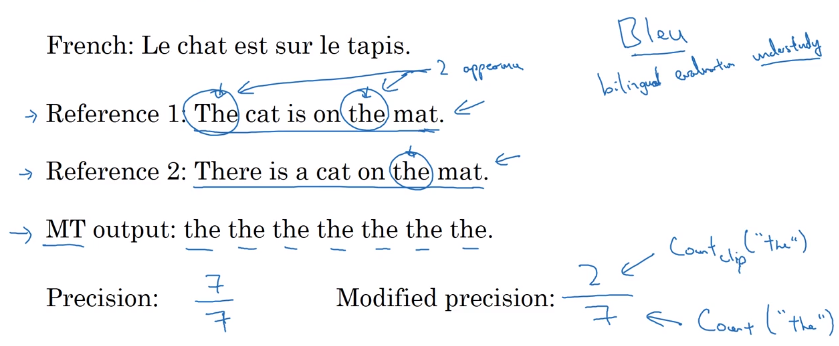Precision on bigrams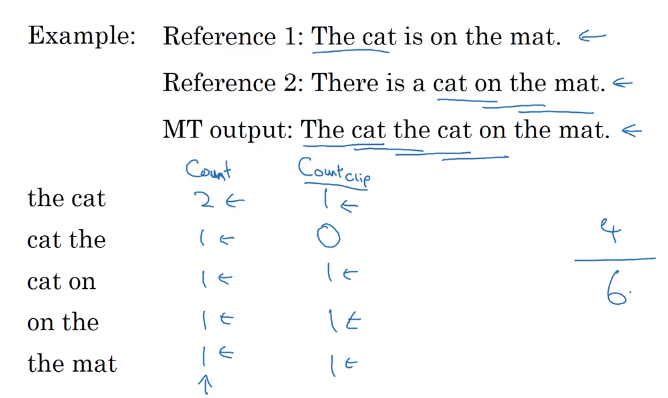For n-grams:
`Pn = sum(count_clip of ngram in yhat) / sum(count of ngram in yhat)`

Bleu score
Combined Bleu score: exp of avearged precision.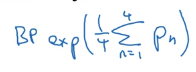BP: brevety penalty (penalize short translations)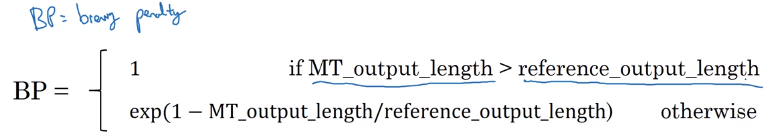### Attention Model Intuition

Human translator: generate translation one part after another, instead of memorize (encode) whole sentence before translate.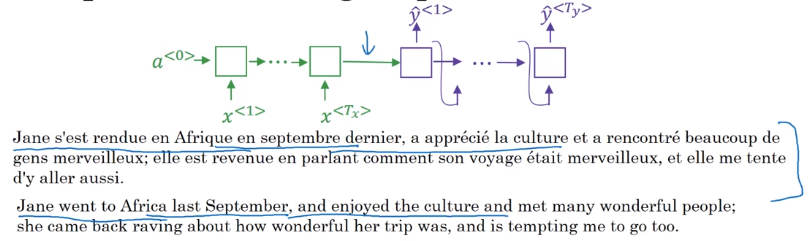• Input: run B-RNN to get hidden features for each word `a<t>`
• Output: also an RNN,

at each step, using context with attention weights `alpha` to focus on only parts of input features.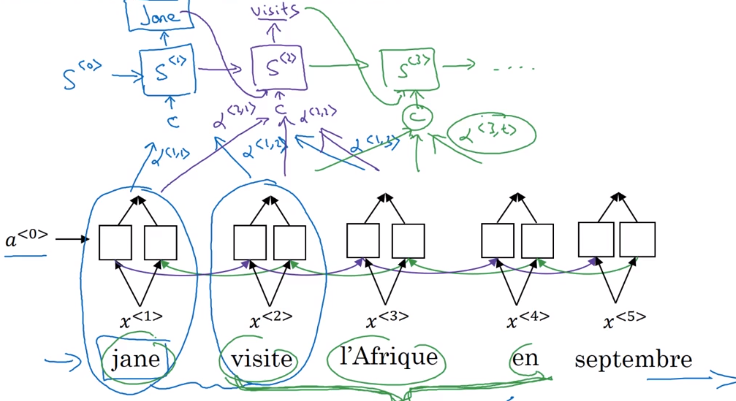Attention `alpha<t,t'>`: how much attention to pay to `t`'th input word when generating `t`th output word: depends on previous output `s<t-1>`, and RNN input feature `a<t'>`.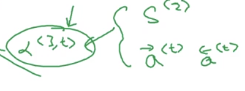### Attention Model

Recap of attention model:
Feature vector at `t`'th input word: `a<t'>`
context: input features, weighted by attention weights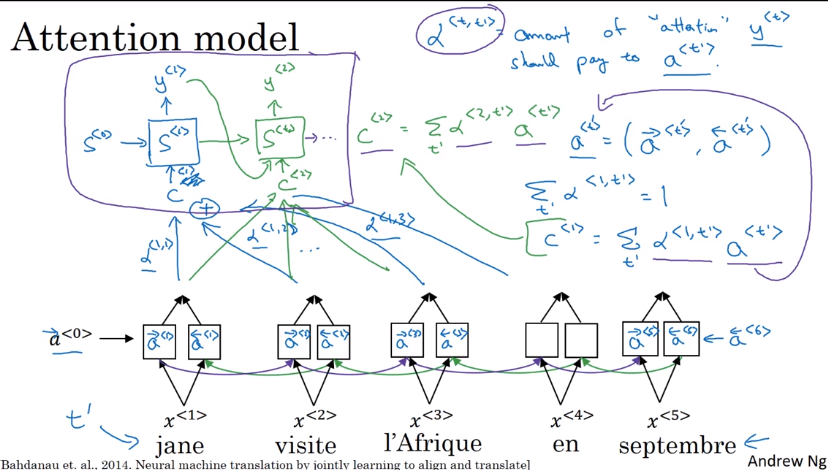Computing attention alpha
Use `a<t,t'>=softmax(e<t,t'>)` to ensure attention is normalized (over all `t`'s) to one.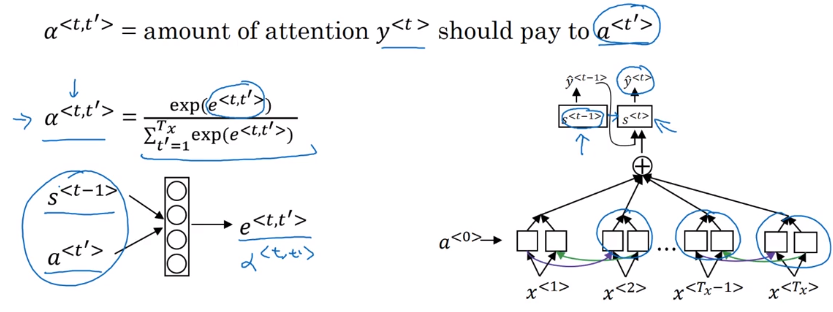⇒ The mapping function from `a<t'>` and `s<t-1>` to attention logits `e<t,t'>` is unknown
→ plug in a NN: `e<t,t'> = W * (s<t-1>, a<t'>)`— and trust backprop !

downside: quadratique time complexity (`Tx * Ty`) → acceptable in MT, since input/output seqs are not that long...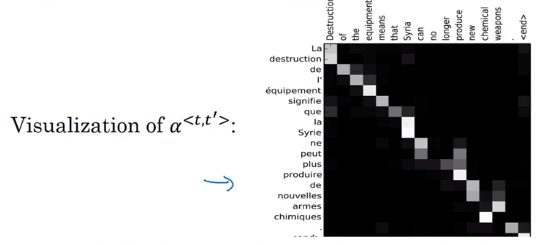## II-Speech recognition - Audio data

### Speech recognition

seq2seq, where x = audio clip / spectrum gram, y=transcript
pre-DL era: phonemes (hand-engineered basic unit of sound) → no longer necessary with end-to-end learning on large dataset.
Dataset: 300~3000 hours

CTC cost : "Connectionist temporal classification".
Pb in speech recogintion with many-to-many RNN: number of input timesteps are much longer than output.
→ blank and repeated characters are considered correct (collapse repeated characters afterwards)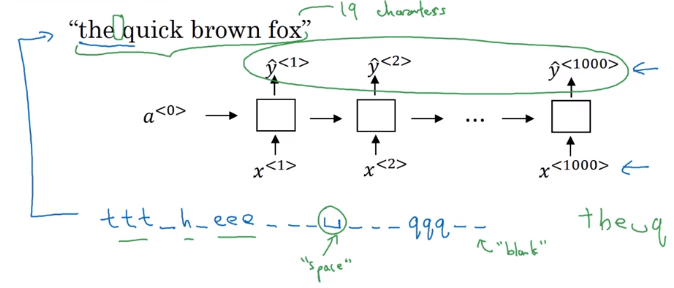### Trigger Word Detection

Train with an RNN.
Data: audio clips
→ set label 1 right after the trigger word.
pb: unbalanced dataset (a lot of 0s) → label = 1 for several timesteps after trigger word.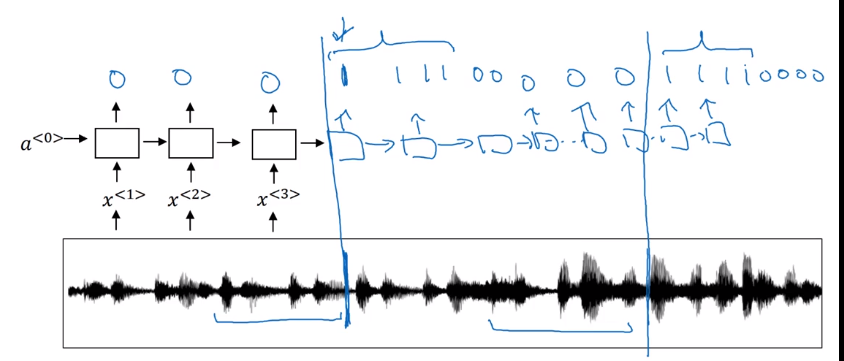## Conclusion and thank you

Deep learning is a super power.